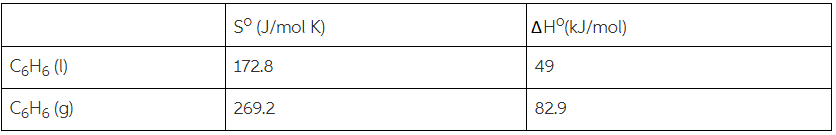# Problem: Calculate the boiling point of benzene, C6H6 from the data given below:C6H6 (l) → C6H6 (g)

###### FREE Expert Solution

(1) Calculating for ΔS°rxn:

ΔS°rxn = 96.4 J/K•mol

90% (154 ratings)###### Problem Details

Calculate the boiling point of benzene, C6H6 from the data given below:

C6H6 (l)  C6H6 (g)